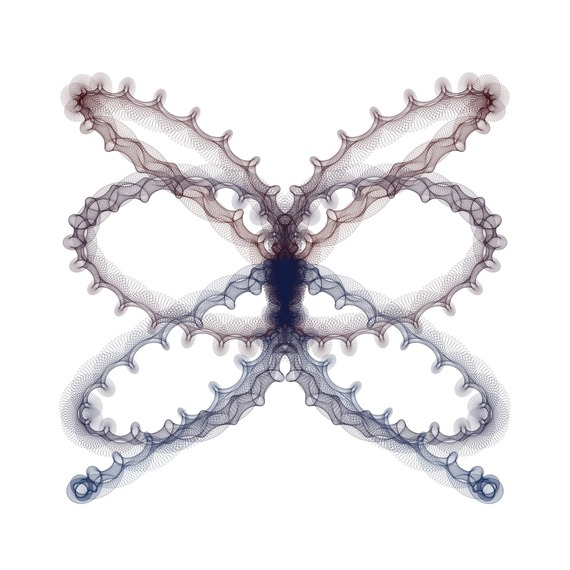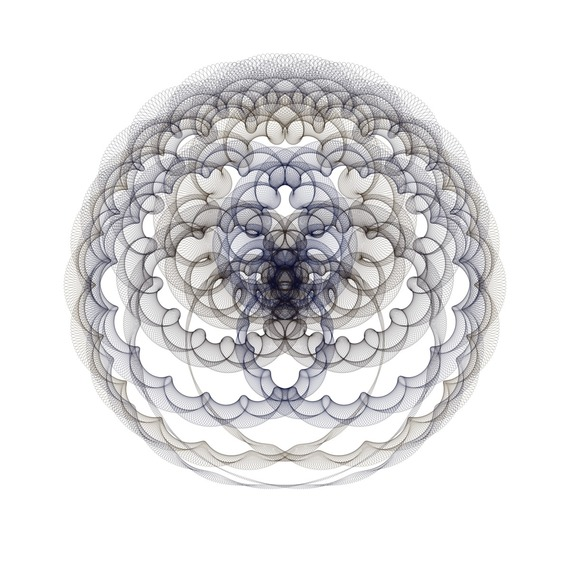# These Beautiful Images Are Created By Drawing Ellipses

We can create an unlimited number of beautiful images by drawing ellipses. The following images are some examples that I have created by some computer programs. At the end of this post you can see the mathematical descriptions of "Butterfly" and "6,000 Ellipses (3)".

ButterflyThis image shows 8,000 ellipses. For each k=1,2,3,...,8000 the foci of the k-th ellipse are:

A(k)+iB(k)+C(k)e^(300πik/8000)

and

A(k)+iB(k)-C(k)e^(300πik/8000)

and the eccentricity of the k-th ellipse is D(k), where

A(k)=(sin(14πk/8000))^3,

B(k)=sin(14πk/8000)sin(10πk/8000),

C(k)=(1/200)+(1/20)+(1/20)cos(22πk/8000),

D(K)=(199/200)-(1/7)(sin(22πk/8000))^8.

6,000 Ellipses (3)This image shows 6,000 ellipses. For each k=1,2,3,...,6000 the foci of the k-th ellipse are:

A(k)+iB(k)+C(k)e^(200πik/6000)

and

A(k)+iB(k)-C(k)e^(200πik/6000)

and the eccentricity of the k-th ellipse is D(k), where

A(k)=cos(16πk/6000)cos(6πk/6000),

B(k)=sin(16πk/6000)cos(6πk/6000),

C(k)=(1/200)+(1/14)+(1/14)(sin(10πk/6000))^3,

D(k)=(49/50)-(1/7)(sin(6πk/6000))^2.

See more images at: mathematics.culturalspot.org.